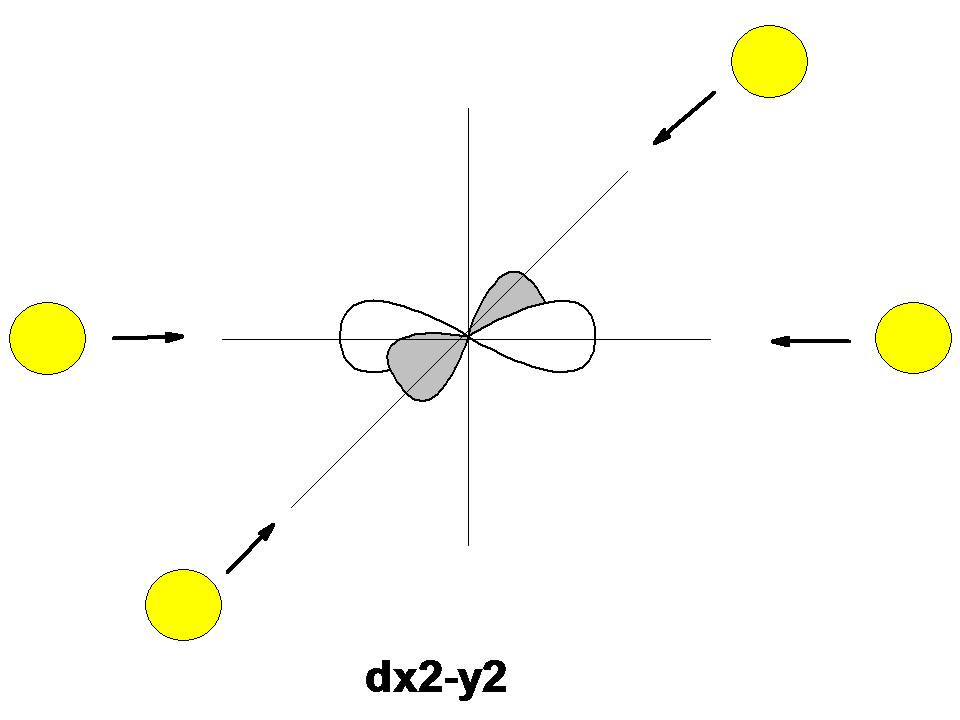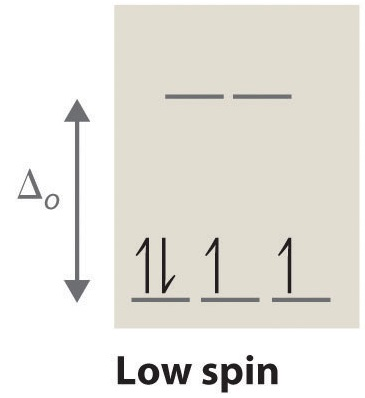# High Spin and Low Spin Complexes

In a tetrahedral complex, Δt is relatively small even with strong-field ligands as there are fewer ligands to bond with. It is rare for the Δt of tetrahedral complexes to exceed the pairing energy. Usually, electrons will move up to the higher energy orbitals rather than pair. Because of this, most tetrahedral complexes are high spin.

## Square Planar Complexes

A square planar complex also has a coordination number of 4. The structure of the complex differs from tetrahedral because the ligands form a simple square on the x and y axes. Because of this, the crystal field splitting is also different. Since there are no ligands along the z-axis in a square planar complex, the repulsion of electrons in the dxz, dyz, and the dz2 orbitals are considerably lower than that of the octahedral complex (the dz2 is slightly higher in energy to the "doughnut" that lies on the x,y axis). The dx2-y2 orbital has the most energy, followed by the dxy orbital, which is followed by the remaining orbtails (although dz2 has slightly more energy than the dxz and dyz orbital). This pattern of orbital splitting remains constant throughout all geometries. Whichever orbitals come in direct contact with the ligand fields will have higher energies than orbitals that slide past the ligand field and have more of indirect contact with the ligand fields. So when confused about which geometry leads to which splitting, think about the way the ligand fields interact with the electron orbitals of the central atom.In square planar complexes Δ will almost always be large, even with a weak-field ligand. Electrons tend to be paired rather than unpaired because paring energy is usually much less than Δ. Therefore, square planar complexes are usually low spin.

Tips For Determining High Spin or Low Spin Configurations

1. Determine the shape of the complex (i.e. octahedral, tetrahedral, square planar)
2. Determine the oxidation state of the metal center
3. Determine the d electron configuration of the metal center
4. Draw the crystal field diagram of the complex with regards to its geometry
5. Determine whether the splitting energy is greater than the pairing energy
6. Determine the strength of the ligand (i.e. spectrochemical series)

## Crystal Field Splitting Electron Count

In order to make a crystal field diagram of a particular coordination compound, one must consider the number of electrons. This can be done simply by recognizing the ground state configuration of the electron and then adjusting the number of electrons with respect to the charge of the metal. For example, one can consider the following chemical compounds. These four examples demonstrate how the number of electrons are determined and used in making Crystal Field Diagrams.

Example 4

What is the number of electrons of the metal in this complex: [CoF6]3- ?

SOLUTION

This coordination compound has Cobalt as the central transition metal and 6 Fluoro monodentate ligands. However, in this example as well as most other examples, we will focus on the central transition metal. We must determine the oxidation state of Cobalt in this example. Fluorine has a charge of -1 and the overall molecule has a charge of -3. Since there are six fluorines, the overall charge of fluorine is -6. The charge of Cobalt will add to this -6, so that the charge of the overall molecule is -3. Thus, we know that Cobalt must have a charge of +3 (see below). The electron configuration of Cobalt is [Ar]4s23d7. When observing Cobalt 3+, we know that Cobalt must lose three electrons. The first two to go are from the 4s orbital and Cobalt becomes:[Ar]4s03d7. Then, the next electron leaves the 3d orbital and the configuration becomes: [Ar]4s03d6. Thus, we can see that there are six electrons that need to be apportioned to Crystal Field Diagrams. The pairing of these electrons depends on the ligand. Since Fluorine is a weak field, it will be a high spin complex.

Cobalt charge Fluorine charge Overall charge
x + -1(6) = -3

x + -6 = -3

x=-3+6

x=+3

Example 5

What is the number of electrons of the metal in this complex: [Co(NH3)6]3+?

SOLUTION

This coordination compound has Cobalt as the central Transition Metal and 6 Ammonias as Monodentate Ligands. We must determine the oxidation state of Cobalt in this example. Ammonia has a charge of 0 and the overall molecule has a charge of +3. Since there are six Ammonias the overall charge of of it is 0. The charge of Cobalt will add to this 0, so that the charge of the overall molecule is +3. Thus, we know that Cobalt must have a charge of +3 (see below). In order to find the number of electrons, we must focus on the central Transition Metal. The electron configuration of Cobalt is [Ar]4s23d7. When observing Cobalt 3+, we know that Cobalt must lose three electrons. The first two to go are from the 4s orbital and Cobalt becomes:[Ar]4s03d7. Then, the next electron leaves the 3d orbital and the configuration becomes: [Ar]4s03d6. Thus, we can see that there are six electrons that need to be apportioned to Crystal Field Diagrams. The pairing of these electrons depends on the ligand. Since Ammonia is a strong field ligand, it will be a low spin complex.

Cobalt charge Ammonia charge Overall charge
x + 0(6) = +3

x + 0 = +3

x=+3

Example 6

What is the number of electrons of the metal in this complex: [Fe(CN)6]3-?

SOLUTION

This coordination compound has Iron as the central Transition Metal and 6 Cyanides as Monodentate Ligands. We must determine the oxidation state of Iron in this example. Cyanide has a charge of -1 and the overall molecule has a charge of -3. Since there are six Cyanides the overall charge of of it is -6. The charge of Iron will add to this -6, so that the charge of the overall molecule is -3. Thus, we know that Iron must have a charge of +3 (see below). In order to find the number of electrons, we must focus on the central Transition Metal. The electron configuration of Iron is [Ar]4s23d6. When observing Iron 3+, we know that Iron must lose three electrons. The first two to go are from the 4s orbital and Iron becomes:[Ar]4s03d6. Then, the next electron leaves the 3d orbital and the configuration becomes: [Ar]4s03d5. Thus, we can see that there are five electrons that need to be apportioned to Crystal Field Diagrams. The pairing of these electrons depends on the ligand. Since Cyanide is a strong field ligand, it will be a low spin complex.

Iron charge Cyanide charge Overall charge
x + -1(6) = -3

x + -6 = -3

x=-3+6

x=+3

Example 7

[Ni(CN)4]2-

SOLUTION

This coordination compound has Nickel as the central Transition Metal and 4 Cyanides as Monodentate Ligands. We must determine the oxidation state of Nickel in this example. Cyanide has a charge of -1 and the overall molecule has a charge of -2. Since there are four Cyanides, the overall charge of it is -4. The charge of Nickel will add to this -4, so that the charge of the overall molecule is -2. Thus, we know that Nickel must have a charge of +2 (see below). In order to find the number of electrons, we must focus on the central transition metal. The electron configuration of Nickel is [Ar]4s23d8. When observing Nickel 3+, we know that Nickel must lose two electrons. The two to go are from the 4s orbital and Nickel becomes:[Ar]4s03d8. Thus, we can see that there are eight electrons that need to be apportioned to Crystal Field Diagrams. The pairing of these electrons depends on the ligand. Since Cyanide is a strong field ligand, it will be a low spin complex.

Nickel charge Cyanide charge Overall charge
x + -1(4) = -2

x + -4 = -2

x=-2+4

x=+2

## Applications

### Magnetism

The crystal field splitting can also be used to figure out the magnetism of a certain coordination compound. Recall, that diamagnetism is where all the electrons are paired and paramagnetism is where one or more electron is unpaired. This property can be used to determine the magnetism and in some cases the filling of the orbitals. For example, given a high spin octahedral molecule, one just has to fill in all the orbitals and check for unpaired electrons. If no unpaired electrons exist, then the molecule is diamagnetic but if unpaired molecules do exist, the molecule is paramagnetic. These properties of magnetism can also be used to predict how the orbitals will be filled, an alternate method to relying on spin to predict the filling of orbitals. For example, if a given molecule is diamagnetic, the pairing must be done in such a way that no unpaired electrons exist. On the other hand, if the given molecule is paramagnetic, the pairing must be done in such a way that unpaired molecules do exist. Examples of these properties and applications of magnetism are provided below.

### Color

Crystal field splitting can be used to account for the different colors of the coordinate compounds. Low spin complexes with strong field ligands absorb light at shorter wavelengths (higher energy) and high spin complexes with weak field ligands absorb light at longer wavelengths (lower energy). Based on the ligands involved in the coordination compound, the color of that coordination compound can be estimated using the strength the ligand field. See Tanabe-Sugano Diagrams for more advanced applications.

## Sample Problems

### Questions

1. Is square planar usually low spin or high spin?
2. Draw the crystal field energy diagram of [Cu(Cl)6]-4.
3. What is the spectrochemical series?
4. Draw the crystal field energy diagram of [Mn(CN)4]-1.
5. What causes the energy difference between the orbitals in an octahedral field?
6. Classify the spin of [FeBr4]-2.

1. Due to the high crystal field splitting energy, square planar complexes are usually low spin.
2. By doing some simple algebra and using the -1 oxidation state of chloro ligand and the overall charge of -4, we can figure out that the oxidation state of copper is +2 charge. In its non-ionized state, copper has the following electron distribution: [Ar]4s13d10. +2 means that copper will loose two electrons and will thus have a configuration of [Ar]3d9. Using the spectrochemical series, we can figure out that the chloro ligand is a weak field and induces low spin complex. How this is meaningless this problem since the appropriate crystal field energy diagram shows that only one configuration is possible irrespective of the strength of the ligand field:1. The spectrochemical series is a series that orders ligands based on their field strength. It is often used in problems to determine the strength and spin of a ligand field so that the electrons can be distributed appropriately.
2. Just like problem 2, the first thing to do is to figure out the charge of Mn. Since we know the CN has a charge of -1, and there are four of them, and since the overall molecule has a charge of -1, manganese has a oxidation state of +3. In its ground state, manganese has the following electron distribution: [Ar]4s23d5. The +3 oxidation state means that manganese will loose three electrons and will thus have a configuration of [Ar]3d4. Using the spectrochemical series, we can figure out the strength of the CN ligand field, which is a strong field ligand and induces low spin complexes. The appropriate crystal field energy diagram would look like:1. The negative-negative repulsion between the electrons of the central atom and between the ligand field causes certain orbitals, namely the dz2 and dx2-y2 orbitals, to have higher energy than the dxz, dxy, and dyz orbitals.
2. Since the bromo ligand is a weak field ligand (as per the spectrochemical series), this molecule is high spin.